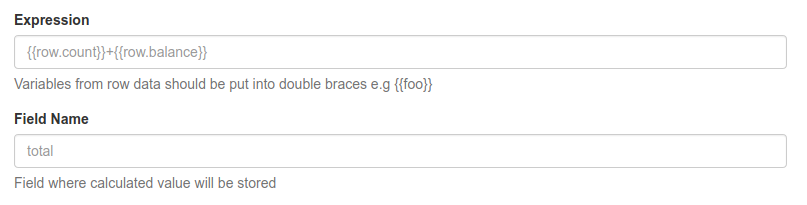# Overview

Evaluates mathematic function and put value into new field## Description

This function performs a mathematical action on the specified fields in each event, and saves the result in a new field in the original event. You can set any mathematical expression in the function parameters. To access event fields, use double brackets. For example, to calculate the sum of two fields in each event, you can specify a mathematical action as {{row.field1}} + {{as.field2}}
If the specified field is not present in the event, its value is 0.

## Function Schema## Examples

For example, each event has a 'count' and 'price' field. We want to find out the total cost of all product units. To do this, set Expression = '{{count}}*{{price}}' and Field Name = 'total' in the function parameters. As a result, the 'total' field will be added to each event, containing the result of multiplying count and price.